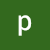We are going to develop a model which can Classify a digit from Mnist and also can classify the addition of predected numbers and a random number.We will Do this task using PyTorch:

# PyTorch Model Building

We have to Build a neural network that can:

1. Take 2 inputs: An image from MNIST data set and arandom number between 0 and 9

2. Gives two outputs: The “number” that was represented by the MNIST image, and the “sum” of this number with the random number that was generated and sent as the input to the network

We can mix fully connected layers and convolution…

# CHAPTER 1

As we are going to understand vision let us talk about Eye first. The biggest question that we can ask is, How eyes are able to see things ? .What is the mechanism behind ? What is happening inside brain to identify the image ?To understand the building blocks of CNN we need to understand this first .

# The Human Eye

Internally our eyes see things by Rods and Cones shown in the figure . Rods are sensitive to low light intensity and able to detect shades of grey (black and white ). Rods can recognise shapes but not colours .where cones are…